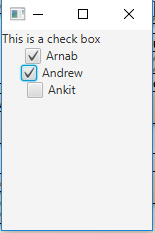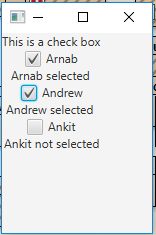Related Articles
JavaFX | Checkbox
• Last Updated : 24 Oct, 2019

CheckBox is a part of JavaFX package. CheckBox is a box with a tick on it when selected and empty when not selected. At first, checkboxes may seem similar to radio buttons, but there exists the difference between them that checkboxes cannot be combined into toggle groups, which means we cannot select multiple options at the same time.

States of CheckBox:

• Checked: When indeterminate is false and checked is true
• Unchecked: When indeterminate is false and checked is false
• Undefined: When indeterminate is true

• Constructor of the class are:

1. CheckBox() : Creates a check box with an empty string for its label.
2. CheckBox(String t) : Creates a check box with the given text as its label.

Commonly used methods:

method explanation
isIndeterminate() Gets the value of the property indeterminate.
isSelected() Gets the value of the property selected.
selectedProperty() Indicates whether this CheckBox is checked.
setIndeterminate(boolean v) Sets the value of the property indeterminate.
setSelected(boolean v) Sets the value of the property selected.

Below programs illustrate the use of CheckBox in JavaFX package:

1. Program to create checkbox and add it to stage: This program creates a multiple CheckBox indicated by the name c. The CheckBox will be created inside a scene, which in turn will be hosted inside a stage. The indeterminate state of the checkbox would be initially set to true using the setIndeterminate() function. The function setTitle() is used to provide title to the stage. Then a tile pane is created, on which addChildren() method is called to attach the CheckBox and the label inside the scene. Finally the show() method is called to display the final results.

 `import` `javafx.application.Application; ` `import` `javafx.scene.Scene; ` `import` `javafx.scene.control.*; ` `import` `javafx.scene.layout.*; ` `import` `javafx.event.ActionEvent; ` `import` `javafx.event.EventHandler; ` `import` `javafx.collections.*; ` `import` `javafx.stage.Stage; ` `public` `class` `Checkbox_1 ``extends` `Application { ` ` `  `    ``// launch the application ` `    ``public` `void` `start(Stage s) ` `    ``{ ` `        ``// set title for the stage ` `        ``s.setTitle(``"creating CheckBox"``); ` ` `  `        ``// create a tile pane ` `        ``TilePane r = ``new` `TilePane(); ` ` `  `        ``// create a label ` `        ``Label l = ``new` `Label(``"This is a check box"``); ` ` `  `        ``// string array ` `        ``String st[] = { ``"Arnab"``, ``"Andrew"``, ``"Ankit"` `}; ` ` `  `        ``// add label ` `        ``r.getChildren().add(l); ` ` `  `        ``for` `(``int` `i = ``0``; i < st.length; i++) { ` ` `  `            ``// create a checkbox ` `            ``CheckBox c = ``new` `CheckBox(st[i]); ` ` `  `            ``// add label ` `            ``r.getChildren().add(c); ` ` `  `            ``// set IndeterMinate ` `            ``c.setIndeterminate(``true``); ` `        ``} ` ` `  `        ``// create a scene ` `        ``Scene sc = ``new` `Scene(r, ``150``, ``200``); ` ` `  `        ``// set the scene ` `        ``s.setScene(sc); ` ` `  `        ``s.show(); ` `    ``} ` ` `  `    ``public` `static` `void` `main(String args[]) ` `    ``{ ` `        ``// launch the application ` `        ``launch(args); ` `    ``} ` `} `

Output:2. Java Program to create check box and add event handler to it: This program creates a multiple CheckBox indicated by the name c. An Event handler will be created to handle the events ( toggle the label associated with textbox to depict the state of checkbox). The event would be set to the checkbox using setOnAction() function. The CheckBox will be created inside a scene, which in turn will be hosted inside a stage. The function setTitle() is used to provide title to the stage. Then a tile pane is created, on which addChildren() method is called to attach the CheckBox and the label inside the scene. Finally, the show() method is called to display the final results.

 `import` `javafx.application.Application; ` `import` `javafx.scene.Scene; ` `import` `javafx.scene.control.*; ` `import` `javafx.scene.layout.*; ` `import` `javafx.event.ActionEvent; ` `import` `javafx.event.EventHandler; ` `import` `javafx.collections.*; ` `import` `javafx.stage.Stage; ` `public` `class` `Checkbox_2 ``extends` `Application { ` ` `  `    ``// launch the application ` `    ``public` `void` `start(Stage s) ` `    ``{ ` `        ``// set title for the stage ` `        ``s.setTitle(``"creating CheckBox"``); ` ` `  `        ``// create a tile pane ` `        ``TilePane r = ``new` `TilePane(); ` ` `  `        ``// create a label ` `        ``Label l = ``new` `Label(``"This is a check box"``); ` ` `  `        ``// string array ` `        ``String st[] = { ``"Arnab"``, ``"Andrew"``, ``"Ankit"` `}; ` ` `  `        ``// add label ` `        ``r.getChildren().add(l); ` ` `  `        ``for` `(``int` `i = ``0``; i < st.length; i++) { ` ` `  `            ``// create a checkbox ` `            ``CheckBox c = ``new` `CheckBox(st[i]); ` ` `  `            ``// add checkbox ` `            ``r.getChildren().add(c); ` ` `  `            ``Label l1 = ``new` `Label(st[i] + ``" not selected"``); ` ` `  `            ``// create a string ` `            ``String s1 = st[i]; ` ` `  `            ``// create a event handler ` `            ``EventHandler event = ``new` `EventHandler() { ` ` `  `                ``public` `void` `handle(ActionEvent e) ` `                ``{ ` `                    ``if` `(c.isSelected()) ` `                        ``l1.setText(s1 + ``" selected "``); ` `                    ``else` `                        ``l1.setText(s1 + ``" not selected "``); ` `                ``} ` ` `  `            ``}; ` ` `  `            ``// set event to checkbox ` `            ``c.setOnAction(event); ` ` `  `            ``// add label ` `            ``r.getChildren().add(l1); ` `        ``} ` ` `  `        ``// create a scene ` `        ``Scene sc = ``new` `Scene(r, ``150``, ``200``); ` ` `  `        ``// set the scene ` `        ``s.setScene(sc); ` ` `  `        ``s.show(); ` `    ``} ` ` `  `    ``public` `static` `void` `main(String args[]) ` `    ``{ ` `        ``// launch the application ` `        ``launch(args); ` `    ``} ` `} `

Output:Attention reader! Don’t stop learning now. Get hold of all the important Java Foundation and Collections concepts with the Fundamentals of Java and Java Collections Course at a student-friendly price and become industry ready.

My Personal Notes arrow_drop_up
Recommended Articles
Page :